• 2020-11-12 23:42:57

本文主要记录一下pytorch里面的二分类及多分类交叉熵损失函数的使用。

import torch
import torch.nn as nn
import torch.nn.functional as F
torch.manual_seed(2020)

<torch._C.Generator at 0x7f4e8b3298b0>


## 二分类交叉熵损失函数

#### Single

m = nn.Sigmoid()
loss = nn.BCELoss()
print(input)
target = torch.empty(3).random_(2)
output = loss(m(input), target)
print(output)
f_output = F.binary_cross_entropy(m(input), target)
print(f_output)
l_output = nn.BCEWithLogitsLoss()(input, target)
print(l_output)

tensor([ 1.2372, -0.9604,  1.5415], requires_grad=True)


#### Batch

m = nn.Sigmoid()
loss = nn.BCELoss()
target = torch.empty(32,5).random_(2)
output = loss(m(input), target)
print(output)
f_output = F.binary_cross_entropy(m(input), target)
print(f_output)
l_output = nn.BCEWithLogitsLoss()(input, target)
print(l_output)

tensor([[ 1.2986,  1.5832, -1.1648,  0.8027, -0.9628],
[-1.5793, -0.2155,  0.4706, -1.2511,  0.7105],
[-0.1274, -1.9361,  0.8374,  0.0081, -0.1504],
[ 0.1521,  1.1443,  0.2171, -1.1438,  0.9341],
[-3.3199,  1.2998,  0.3918,  0.8327,  1.2411],
[-0.8507, -0.1016, -1.2434, -0.5755,  0.1871],
[-0.3064,  1.3751,  1.8478,  0.0326,  0.2032],
[ 0.1782,  2.3037,  1.5948, -1.4731,  1.5312],
[-0.9075, -1.7135,  0.4650, -1.7061,  0.0625],
[-1.1904,  0.1130, -1.6609, -0.2000, -0.1422],
[ 0.3307, -0.8395, -1.3068, -0.8891,  0.9858],
[ 0.5484,  0.7461, -1.0738, -2.2162,  0.6801],
[-0.8803,  0.9934, -1.6438,  0.3860,  0.4111],
[-1.1078, -0.9629, -0.9534, -0.6207,  0.6885],
[-0.0175,  1.9496,  0.9740, -0.4687, -0.6127],
[ 0.3713,  0.8074,  0.3072,  1.1604, -0.2669],
[-0.1773, -0.2787,  0.1926,  0.7492,  0.7492],
[-0.3126, -0.3321, -1.7287, -3.0126,  0.1194],
[ 1.0486, -0.1890, -0.5853,  0.4353,  0.2619],
[ 1.9726, -0.5510, -0.1826, -0.8600, -0.9906],
[ 0.7551,  0.8431, -0.8461, -1.2120,  0.2908],
[-0.0932, -0.7151, -0.0631,  1.7554,  0.7374],
[-0.1494, -0.6990, -0.1666,  2.0430,  1.3968],
[ 0.2280, -0.3187,  1.0309, -0.1067,  1.1622],
[-1.5120, -0.8617,  1.4165, -0.2361, -0.0355],
[-0.8757, -0.6554,  0.1121, -0.1669, -0.2628],
[-0.8023,  0.2305, -1.1792,  0.4314, -0.3653],
[ 0.7487,  0.5358, -0.2677, -0.8128,  0.3029],
[ 1.4439, -0.5677,  0.5564, -0.2485, -0.3281],
[-2.0259,  1.1038,  1.0615,  1.7317, -0.0531],
[ 0.9083, -0.8274,  0.8101, -1.1375, -1.2009],
[ 0.3300, -0.8760,  1.3459, -1.0209, -0.5313]], requires_grad=True)


### Note

• nn.BCELoss()F.binary_cross_entropy 计算结果是等价的，具体两者差距可见PyTorch 中，nn 与 nn.functional 有什么区别？
• nn.BCEWithLogitsLoss: combines a Sigmoid layer and the BCELoss in one single class. This version is more numerically stable than using a plain Sigmoid followed by a BCELoss as, by combining the operations into one layer, we take advantage of the log-sum-exp trick for numerical stability. 至于为什么更稳定，见 https://blog.csdn.net/u010630669/article/details/105599067

• 二分类交叉熵损失函数的input和target的shape是一致的

## 多分类交叉熵损失函数

#### Single

loss = nn.CrossEntropyLoss()
target = torch.empty(3, dtype=torch.long).random_(5)
output = loss(input, target)
print(output)
f_output = F.cross_entropy(input, target)
print(f_output)

tensor(1.7541, grad_fn=<NllLossBackward>)


#### Batch

loss = nn.CrossEntropyLoss()
input = torch.randn(32, 10, 5, requires_grad=True)
target = torch.empty(32, 5, dtype=torch.long).random_(10)
output = loss(input, target)
print(output)
f_output = F.cross_entropy(input, target)
print(f_output)

tensor(2.7944, grad_fn=<NllLoss2DBackward>)


### Note

• nn.CrossEntropyLossF.cross_entropy 计算结果是等价的。两个函数都结合了 LogSoftmax and NLLLoss 运算
• nn.CrossEntropyLoss 的公式为： loss ⁡ ( x ,  class  ) = − log ⁡ ( exp ⁡ ( x [ c l a s s ] ) ∑ j exp ⁡ ( x [ j ] ) ) = − x [ c l a s s ] + log ⁡ ( ∑ j exp ⁡ ( x [ j ] ) ) \operatorname{loss}(\mathrm{x}, \text { class })=-\log \left(\frac{\exp (\mathrm{x}[\mathrm{class}])}{\sum_{\mathrm{j}} \exp (\mathrm{x}[\mathrm{j}])}\right)=-\mathrm{x}[\mathrm{class}]+\log \left(\sum_{\mathrm{j}} \exp (\mathrm{x}[\mathrm{j}])\right) ,这与我们平时见到的多分类交叉熵损失函数有点不同，具体的推导过程见Pytorch里的CrossEntropyLoss详解

参考自：

• https://pytorch.org/docs/stable/generated/torch.nn.CrossEntropyLoss.html?highlight=crossentropyloss#torch.nn.CrossEntropyLoss
• https://pytorch.org/docs/stable/generated/torch.nn.BCELoss.html?highlight=bceloss#torch.nn.BCELoss
• https://www.cnblogs.com/marsggbo/p/10401215.htmlpytorch
更多相关内容
• 在之前的课程中，我们已经完成了从0建立深层神经...接下来，我们将从梯度下降法向外拓展，介绍神经网络的损失函数、常用优化算法等信息，实现神经网络的学习和迭代。本节主要讲解神经网络常用的损失函数，并在PyTorch

## 一、机器学习中的优化思想

在之前的学习中，我们建立神经网络时总是先设定好与的值（或者由我们调用的PyTorch类帮助我们随机生成权重向量），接着通过加和求出 z z ，再在 z z 上嵌套sigmoid或者softmax函数，最终获得神经网络的输出。我们的代码及计算流程，总是从神经网络的左侧向右侧计算的。之前我们提到过，这是神经网络的正向传播过程。但很明显，这并不是神经网络算法的全流程，这个流程虽然可以输出预测结果，但却无法保证神经网络的输出结果与真实值接近。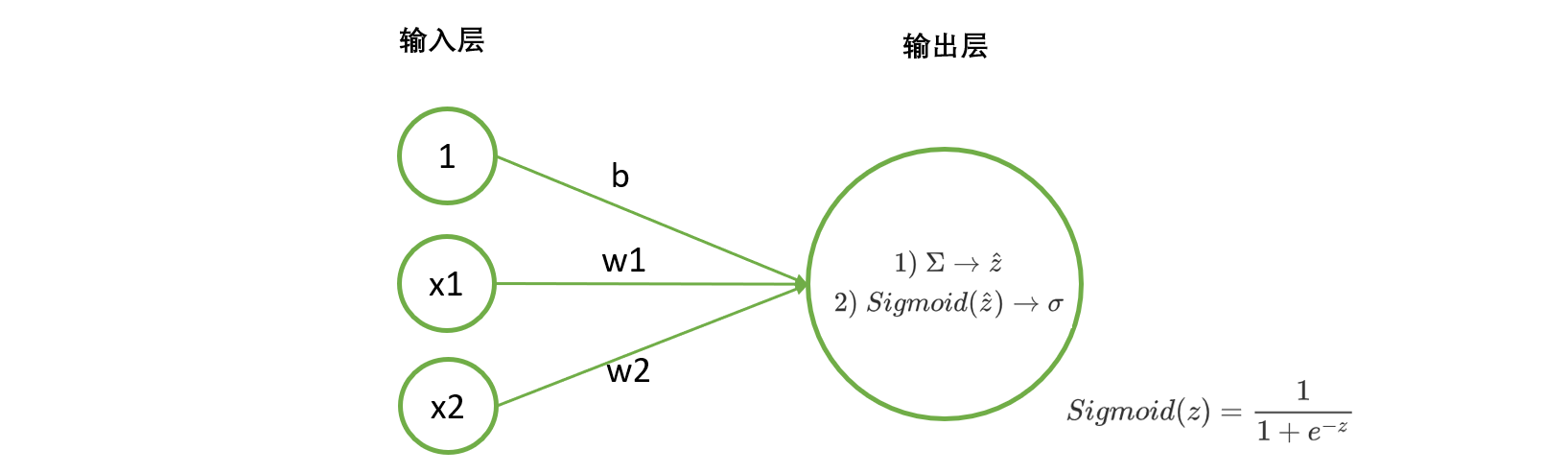在讲解线性回归时，我们提起过，线性回归的任务就是构造一个预测函数来映射输入的特征矩阵 X X 和标签值 y y 的线性关系。构造预测函数核心就是找出模型的权重向量 w w ，并令线性回归的输出结果与真实值相近，也就是求解线性方程组中的 w w b b 。对神经网络而言也是如此，我们的核心任务是求解一组最适合的 w w b b ，令神经网络的输出结果与真实值接近。找寻这个 w w b b 的过程就是“学习”，也叫做“训练”或者“建模”。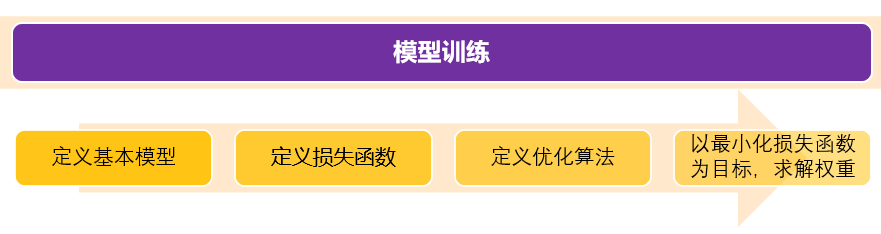1）提出基本模型，明确目标
我们的基本模型就是我们自建的神经网络架构，我们需要求解的就是神经网络架构中的权重向量 w w
2）确定损失函数/目标函数
我们需要定义某个评估指标，用以衡量模型权重为 w w 的情况下，预测结果与真实结果的差异。当真实值与预测值差异越大时，我们就认为神经网络学习过程中丢失了许多信息，丢失的这部分被形象地称为”损失“，因此评估真实值与预测值差异的函数被我们称为“损失函数”。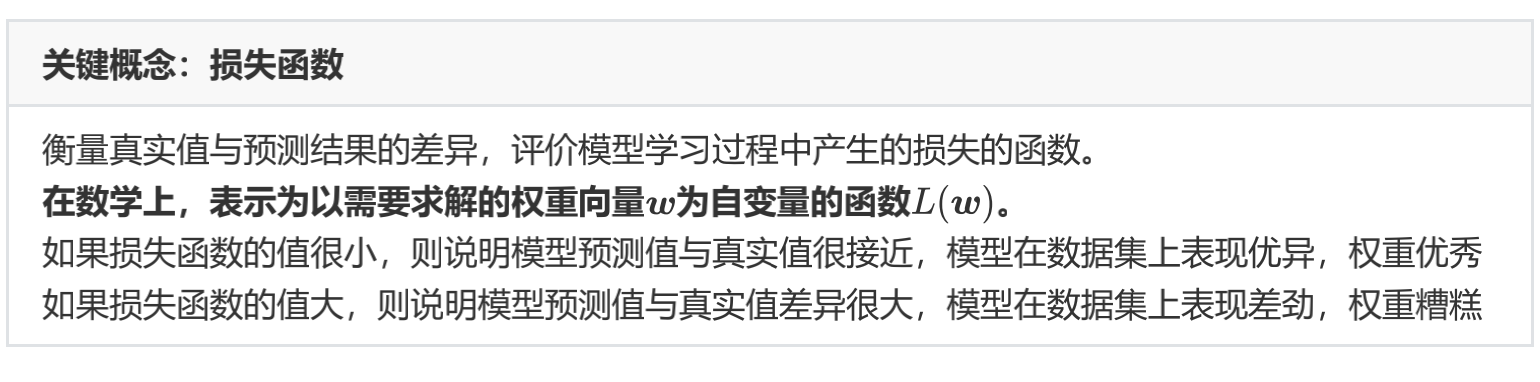我们希望损失函数越小越好，以此，我们将问题转变为求解函数 L ( w ) L(w) 的最小值所对应的自变量 w w 。但是，损失函数往往不是一个简单的函数，求解复杂函数就需要复杂的数学工具。在这里，我们使用的数学工具可能有两部分：

• 将损失函数 L ( w ) L(w) 转变成凸函数的数学方法，常见的有拉格朗日变换等
• 在凸函数上求解 L ( w ) L(w) 的最小值对应的 w w 的方法，也就是以梯度下降为代表的优化算法

3）确定适合的优化算法
4）利用优化算法，最小化损失函数，求解最佳权重（训练）
之前我们在线性回归上走过这个全流程。对线性回归，我们的损失函数是SSE，优化算法是最小二乘法和梯度下降法，两者都是对机器学习来说非常重要的优化算法。但遗憾的是，最小二乘法作为入门级优化算法，有较多的假设和先决条件，不足以应对神经网络需要被应用的各种复杂环境。梯度下降法应用广泛，不过也有很多问题需要改进。接下来，我将主要以分类深层神经网络为例来介绍神经网络中所使用的入门级损失函数及优化算法。

## 二、回归：误差平方和SSE

对于回归类神经网络而言，最常见的损失函数是SSE（Sum of the Squared Errors），现在已经是我们第三次见到SSE的公式了：
S S E = ∑ i = 1 m ( z i − z ^ i ) 2 S S E=\sum_{i=1}^{m}\left(z_{i}-\hat{z}_{i}\right)^{2}
其中 z i z_{i} 是样本 i i 的真实值，而 z ^ i \hat{z}_{i} 是样本 i i 的预测值。对于全部样本的平均损失，则可以写作：
M S E = 1 m ∑ i = 1 m ( z i − z ^ i ) 2 M S E=\frac{1}{m} \sum_{i=1}^{m}\left(z_{i}-\hat{z}_{i}\right)^{2}
在PyTorch中，我们可以简单通过以下代码调用MSE：

import torch
from torch.nn import MSELoss #类
yhat = torch.randn(size=(50,),dtype=torch.float32)
y = torch.randn(size=(50,),dtype=torch.float32)
criterion = MSELoss() #实例化
loss = criterion(yhat,y)
loss #没有设置随机数种子，所以每次运行的数字都会不一致
#tensor(1.5714)

#在MSELoss中有重要的参数，reduction
#当reduction = "mean" (默认也是mean)，则输出MSE
#当reduction = "sum"，则输出SSE
criterion = MSELoss(reduction = "mean") #实例化
criterion(yhat,y)
#tensor(1.5714)
criterion = MSELoss(reduction = "sum")
criterion(yhat,y)
#tensor(78.5707)


## 三、二分类交叉熵损失函数

在这一节中，我们将介绍二分类神经网络的损失函数：二分类交叉熵损失函数（Binary Cross Entropy Loss），也叫做对数损失（log loss）。这个损失函数被广泛地使用在任何输出结果是二分类的神经网络中，即不止限于单层神经网络，还可被拓展到多分类中，因此理解二分类交叉熵损失是非常重要的一环。大多数时候，除非特殊声明为二分类，否则提到交叉熵损失，我们会默认算法的分类目标是多分类。

二分类交叉熵损失函数是由极大似然估计推导出来的，对于有m个样本的数据集而言，在全部样本上的平均损失写作：
L ( w ) = − ∑ i = 1 m ( y i ∗ ln ⁡ ( σ i ) + ( 1 − y i ) ∗ ln ⁡ ( 1 − σ i ) ) L(w)=-\sum_{i=1}^{m}\left(y_{i} * \ln \left(\sigma_{i}\right)+\left(1-y_{i}\right) * \ln \left(1-\sigma_{i}\right)\right)
在单个样本的损失写作：
L ( w ) i = − ( y i ∗ ln ⁡ ( σ i ) + ( 1 − y i ) ∗ ln ⁡ ( 1 − σ i ) ) L(w)_{i}=-\left(y_{i} * \ln \left(\sigma_{i}\right)+\left(1-y_{i}\right) * \ln \left(1-\sigma_{i}\right)\right)
其中，ln是以自然底数 e e 为底的对数函数， w w 表示求解出来的一组权重（在等号的右侧， w w σ \sigma 里），m是样本的个数， y i y_{i} 是样本i上真实的标签， σ i \sigma_{i} 是样本i上，基于参数 w w 计算出来的sigmoid函数的返回值， x i x_{i} 是样本i各个特征的取值。我们的目标，就是求解出使 L ( w ) L(w) 最小的 w w 取值。注意，在神经网络中，特征张量 X X 是自变量，权重是 w w 。但在损失函数中，权重 w w 是损失函数的自变量，特征x和真实标签y都是已知的数据，相当于是常数。不同的函数中，自变量和参数各有不同，因此大家需要在数学计算中，尤其是求导的时候避免混淆。

### 1 极大似然估计求解二分类交叉熵损失

二分类交叉熵损失函数是怎么来的呢？为什么这个函数就能够代表二分类的时候，真实值与预测值的差异呢？

在这里，我们基于极大似然法来推导交叉熵损失，这个推导过程能够帮助我们充分了解交叉熵损失的含义，以及为什么的最小化能够实现模型在数据集上的拟合最好。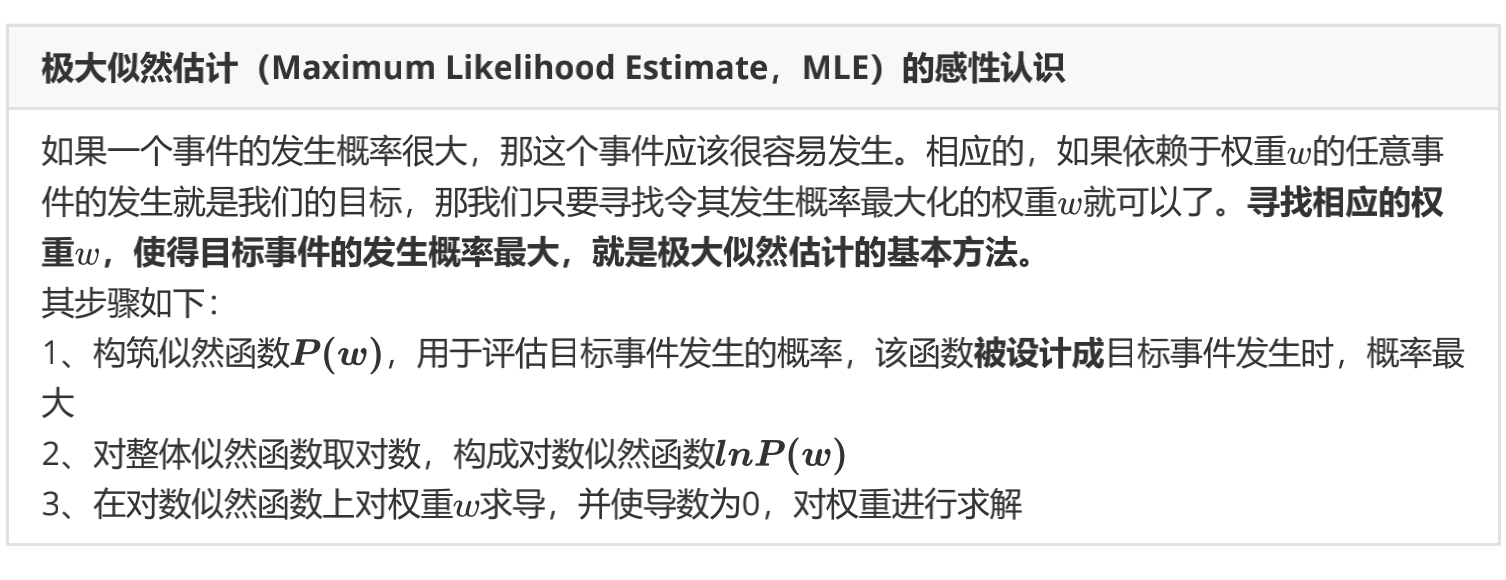在二分类的例子中，我们的“任意事件”就是每个样本的分类都正确，对数似然函数的负数就是我们的损失函数。接下来，我们来看看逻辑回归的对数似然函数是怎样构筑的。

• 构筑对数似然函数

二分类神经网络的标签是[0,1]，此标签服从伯努利分布(即0-1分布)，因此可得：

样本i在由特征向量 x i x_{i} 和权重向量 w w 组成的预测函数中，样本标签被预测为1的概率为：对二分类而言， σ \sigma 就是sigmoid函数的结果。
样本i在由特征向量 x i x_{i} 和权重向量 w w 组成的预测函数中，样本标签被预测为0的概率为：P 1 P_{1} 值为1的时候，代表样本i的标签被预测为1，当 P 0 P_{0} 的值为1的时候，代表样本i的标签被预测为0。 P 0 P_{0} P 1 P_{1} 相加是一定等于1的。

假设样本i的真实标签 y i y_{i} 为1，并且 P 1 P_{1} 也为1的话，那就说明我们将 i i 的标签预测为1的概率很大，与真实值一致，那模型的预测就是准确的，拟合程度很高，信息损失很少。相反，如果真实标签为1，我们的 P 1 P_{1} 却很接近0，这就说明我们将 i i 的标签预测为1的概率很小，即与真实值一致的概率很小，那模型的预测就是失败的，拟合程度很低，信息损失很多。当 y i y_{i} 为0时，也是同样的道理。所以，当 y i y_{i} 为1的时候，我们希望 P 1 P_{1} 非常接近1，当 y i y_{i} 为0的时候，我们希望 P 0 P_{0} 非常接近1，这样，模型的效果就很好，信息损失就很少。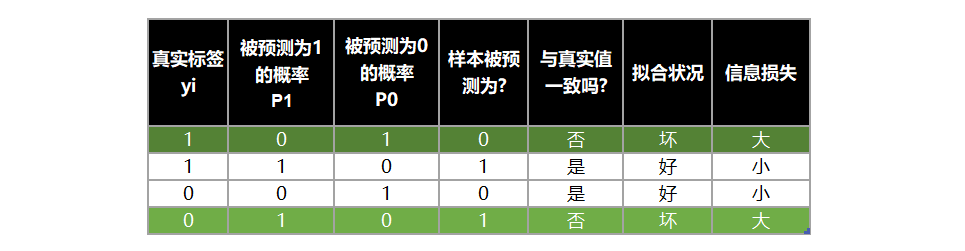将两种取值的概率整合，我们可以定义如下等式：
P ( y ^ i ∣ x i , w ) = P 1 y i ∗ P 0 1 − y i P\left(\hat{y}_{i} \mid \boldsymbol{x}_{i}, \boldsymbol{w}\right)=P_{1}^{y_{i}} * P_{0}^{1-y_{i}}
这个等式代表同时代表了 P 1 P_{1} P 0 P_{0} ，在数学上，它被叫做逻辑回归的假设函数。

当样本i的真实标签 y i y_{i} 为1的时候，1 - y i y_{i} 就等于0， P 0 P_{0} 的0次方就是1，所以 P ( y ^ i ∣ x i , w ) P\left(\hat{y}_{i} \mid \boldsymbol{x}_{i}, \boldsymbol{w}\right) 就等于 P 1 P_{1} ，这个时候，如果 P 1 P_{1} 为1，模型的效果就很好，损失就很小。

同理，当 y i y_{i} 为0的时候， P ( y ^ i ∣ x i , w ) P\left(\hat{y}_{i} \mid \boldsymbol{x}_{i}, \boldsymbol{w}\right) 就等于 P 0 P_{0} ，此时如果 P 0 P_{0} 非常接近1，模型的效果就很好，损失就很小。

所以，为了达成让模型拟合好，损失小的目的，我们每时每刻都希望 P ( y ^ i ∣ x i , w ) P\left(\hat{y}_{i} \mid \boldsymbol{x}_{i}, \boldsymbol{w}\right) 的值等于1。而 P ( y ^ i ∣ x i , w ) P\left(\hat{y}_{i} \mid \boldsymbol{x}_{i}, \boldsymbol{w}\right) 的本质是样本i由特征向量 x i x_{i} 和权重 w w 组成的预测函数中，预测出所有可能的 y ^ i \hat{y}_{i} 的概率，因此1是它的最大值。也就是说，每时每刻，我们都在追求 P ( y ^ i ∣ x i , w ) P\left(\hat{y}_{i} \mid \boldsymbol{x}_{i}, \boldsymbol{w}\right) 的最大值。而寻找相应的参数 w w ，使得每次得到的预测概率最大，正是极大似然估计的基本方法，不过 P ( y ^ i ∣ x i , w ) P\left(\hat{y}_{i} \mid \boldsymbol{x}_{i}, \boldsymbol{w}\right) 是对单个样本而言的，因此我们还需要将其拓展到多个样本上。

P ( y ^ i ∣ x i , w ) P\left(\hat{y}_{i} \mid \boldsymbol{x}_{i}, \boldsymbol{w}\right) 是对单个样本i而言的函数，对一个训练集的m个样本来说，我们可以定义如下等式来表达所有样本在特征张量 X X 和权重向量 w w 组成的预测函数中，预测出所有可能的 y ^ i \hat{y}_{i} 的概率P为：
P = ∏ i = 1 m P ( y ^ i ∣ x i , w ) = ∏ i = 1 m ( P 1 y i ∗ P 0 1 − y i ) = ∏ i = 1 m ( σ i y i ∗ ( 1 − σ i ) 1 − y i ) \begin{aligned} \boldsymbol{P} &=\prod_{i=1}^{m} P\left(\hat{y}_{i} \mid x_{i}, w\right) \\ &=\prod_{i=1}^{m}\left(P_{1}^{y_{i}} * P_{0}^{1-y_{i}}\right) \\ &=\prod_{i=1}^{m}\left(\sigma_{i}^{y_{i}} *\left(1-\sigma_{i}\right)^{1-y_{i}}\right) \end{aligned}
这个函数就是逻辑回归的似然函数。对该概率P取以e为底的对数，再由 log ⁡ ( A ∗ B ) = log ⁡ A + log ⁡ B \log (A * B)=\log A+\log B log ⁡ A B = B log ⁡ A \log A^{B}=B \log A 可得到逻辑回归的对数似然函数：
ln ⁡ P = ln ⁡ ∏ i = 1 m ( σ i y i ∗ ( 1 − σ i ) 1 − y i ) = ∑ i = 1 m ln ⁡ ( σ i y i ∗ ( 1 − σ i ) 1 − y i ) = ∑ i = 1 m ( ln ⁡ σ i y i + ln ⁡ ( 1 − σ i ) 1 − y i ) = ∑ i = 1 m ( y i ∗ ln ⁡ ( σ i ) + ( 1 − y i ) ∗ ln ⁡ ( 1 − σ i ) ) \begin{aligned} \ln \boldsymbol{P} &=\ln \prod_{i=1}^{m}\left(\sigma_{i}^{y_{i}} *\left(1-\sigma_{i}\right)^{1-y_{i}}\right) \\ &=\sum_{i=1}^{m} \ln \left(\sigma_{i}^{y_{i}} *\left(1-\sigma_{i}\right)^{1-y_{i}}\right) \\ &=\sum_{i=1}^{m}\left(\ln \sigma_{i}^{y_{i}}+\ln \left(1-\sigma_{i}\right)^{1-y_{i}}\right) \\ &=\sum_{i=1}^{m}\left(y_{i} * \ln \left(\sigma_{i}\right)+\left(1-y_{i}\right) * \ln \left(1-\sigma_{i}\right)\right) \end{aligned}
这就是我们的二分类交叉熵函数。为了数学上的便利以及更好地定义”损失”的含义，我们希望将极大值问题转换为极小值问题，因此我们对 l n P lnP 取负，并且让权重 w w 作为函数的自变量，就得到了我们的损失函数 ：
L ( w ) = − ∑ i = 1 m ( y i ∗ ln ⁡ ( σ i ) + ( 1 − y i ) ∗ ln ⁡ ( 1 − σ i ) ) L(w)=-\sum_{i=1}^{m}\left(y_{i} * \ln \left(\sigma_{i}\right)+\left(1-y_{i}\right) * \ln \left(1-\sigma_{i}\right)\right)
现在，我们已经将模型拟合中的“最小化损失”问题，转换成了对函数求解极值的问题。这就是一个，基于逻辑回归的返回值 σ i \sigma_{i} 的概率性质以及极大似然估计得出的损失函数。在这个函数上，我们只要追求最小值，就能让模型在训练数据上的拟合效果最好，损失最低

在极大似然估计中，我们只要在对数似然函数上对权重 w w 求导，再令导数为0，就可以求解出最合适的 w w ，但是对于像交叉熵这样复杂的损失函数，加上神经网络中复杂的权重组合，令所有权重的导数为0并一个个求解方程的难度很大。因此我们要使用优化算法，这部分我们下一章展开来聊。

### 2 用tensor实现二分类交叉熵损失

现在，让我们在PyTorch中来实现二分类交叉熵损失函数。首先使用基本的tensor方法来试试看，以加深我们对二分类交叉熵损失的印象：

import torch
import time
N = 3*pow(10,3)
torch.random.manual_seed(420)
X = torch.rand((N,4),dtype=torch.float32)
y = torch.randint(low=0,high=2,size=(N,1),dtype=torch.float32) #high取不到
zhat = torch.mm(X,w)
sigma = torch.sigmoid(zhat)
Loss = -(1/N)*torch.sum((1-y)*torch.log(1-sigma)+y*torch.log(sigma)) #底数默认为e
Loss


注意，在写损失函数这样的复杂函数时，除了普通的加减乘除以外的全部计算，都要使用torch中的函数，因为tensor的运算速度是远远超过普通Python代码，甚至是NumPy的。你可以试着比较在样本量为300W时，以下两行代码运行的时间差异：

#你可以试着比较在样本量为300W时，以下两行代码运行的时间差异。这段代码不需要GPU。
#如果你的电脑内存或计算资源有限，可以试着将样本量调小为30W或3W
N = 3*pow(10,6)
torch.random.manual_seed(420)
X = torch.rand((N,4),dtype=torch.float32)
y = torch.randint(low=0,high=2,size=(N,1),dtype=torch.float32)
zhat = torch.mm(X,w)
sigma = torch.sigmoid(zhat)

start = time.time()
L1 = -(1/N)*torch.sum((1-y)*torch.log(1-sigma)+y*torch.log(sigma))
now = time.time() #seconds
print(now - start)
#0.03389596939086914

start = time.time()
L2 = -(1/N)*sum((1-y)*torch.log(1-sigma)+y*torch.log(sigma))
now = time.time() #seconds
print(now - start)
#11.579372882843018


从运行结果来看，除了加减乘除，我们应该尽量避免使用任何Python原生的计算方法。如果可能的话，让PyTorch处理一切。

### 3 用PyTorch中的类实现二分类交叉熵损失

在PyTorch当中，我们有多种方式可以调用二分类交叉熵损失函数。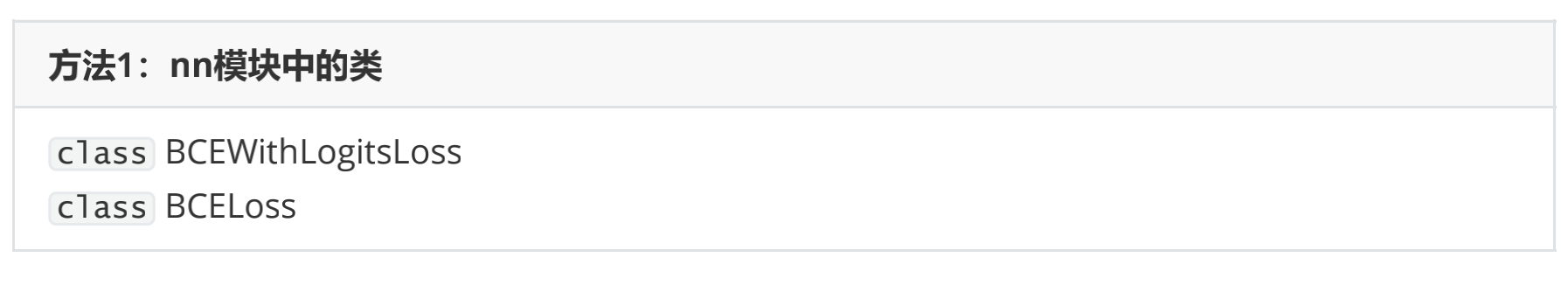对于二分类交叉熵损失，nn提供了两个类：BCEWithLogitsLoss以及BCELoss。虽然PyTorch官方没有直接明确，但实际上两个函数所需要输入的参数不同。

BCEWithLogitsLoss内置了sigmoid函数与交叉熵函数，它会自动计算输入值的sigmoid值，因此需要输入zhat与真实标签，且顺序不能变化，zhat必须在前。

相对的，BCELoss中只有交叉熵函数，没有sigmoid层，因此需要输入sigma与真实标签，且顺序不能变化。

同时，这两个函数都要求预测值与真实标签的数据类型以及结构（shape）必须相同，否则运行就会报错。

接下来，我们来看看这两个类是如何使用的：

import torch.nn as nn
#调用nn模块下的类
criterion = nn.BCELoss() #实例化
loss = criterion(sigma,y) #真实标签在后
loss

criterion2 = nn.BCEWithLogitsLoss() #实例化
loss = criterion2(zhat,y) #真实标签在后
loss


可以看出，两个类的结果是一致的。根据PyTorch官方的公告，他们更推荐使用BCEWithLogitsLoss这个内置了sigmoid函数的类。内置的sigmoid函数可以让精度问题被缩小（因为将指数运算包含在了内部），以维持算法运行时的稳定性，即是说当数据量变大、数据本身也变大时，BCELoss类产生的结果可能有精度问题。所以，当我们的输出层使用sigmoid函数时，我们就可以使用BCEWithLogitsLoss作为损失函数

与MSELoss相同，二分类交叉熵的类们也有参数reduction，默认是”mean“，表示求解所有样本平均的损失，也可换为”sum”，要求输出整体的损失。以及，还可以使用选项“none”，表示不对损失结果做任何聚合运算，直接输出每个样本对应的损失矩阵。

criterion2 = nn.BCEWithLogitsLoss(reduction = "mean")
loss = criterion2(zhat,y)
loss

criterion2 = nn.BCEWithLogitsLoss(reduction = "sum")
loss = criterion2(zhat,y)
loss

criterion2 = nn.BCEWithLogitsLoss(reduction = "none")
loss = criterion2(zhat,y)
loss
#tensor([[1.3102],
#        [0.3155],
#        [0.4247],
#        ...,
#        [0.1727],
#        [0.1716],


第二种方法很少用，我们了解一下即可：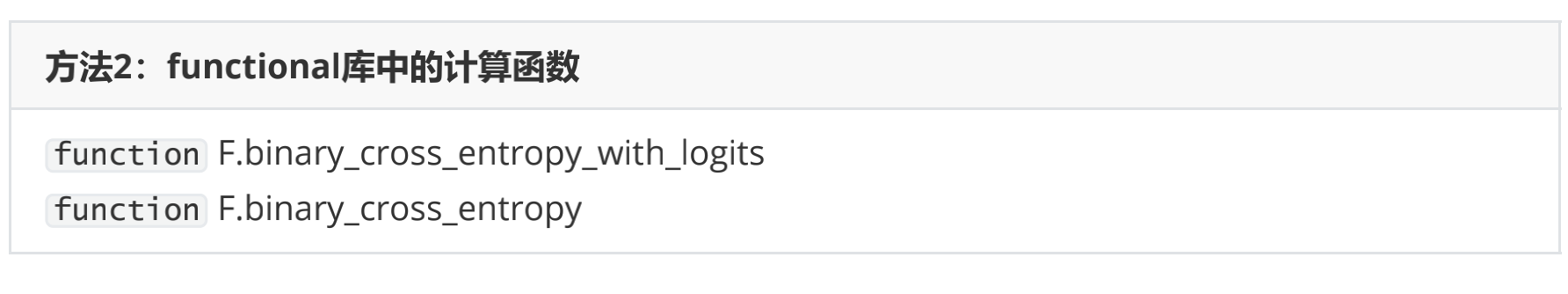和nn中的类们相似，名称中带有Logits的是内置了sigmoid功能的函数，没有带Logits的，是只包含交叉熵损失的函数。对于含有sigmoid功能的函数，我们需要的输入是zhat与标签，不含sigmoid的函数我们则需要输入sigma与标签。同样的，这两个函数对输入有严格的要求，输入的预测值必须与标签结构一致、数据类型一致。我们来看看他们的运行结果：

from torch.nn import functional as F #直接调用functional库中的计算函数
F.binary_cross_entropy_with_logits(zhat,y)

F.binary_cross_entropy(sigma,y)


在这里，两个函数的运行结果是一致的。同样的，PyTorch官方推荐的是内置sigmoid功能的函数binary_cross_entropy_with_logits。通常来说，我们都使用类，不使用函数。虽然代码会因此变得稍稍有点复杂，但为了代码的稳定性与日后维护，使用类是更好的选择。当然，在进行代码演示和快速测算的时候，使用函数或者类都没有问题。

## 四、多分类交叉熵损失函数

### 1 由二分类推广到多分类

二分类交叉熵损失可以被推广到多分类上，但在实际处理时，二分类与多分类却有一些关键的区别。依然使用极大似然估计的推导流程，首先我们来确定单一样本概率最大化后的似然函数。

对于多分类的状况而言，标签不再服从伯努利分布（0-1分布），因此我们可以定义，样本i在由特征向量 x i x_{i} 和权重向量 w w 组成的预测函数中，样本标签被预测为类别k的概率为：
P k = P ( y ^ i = k ∣ x i , w ) = σ P_{k}=P\left(\hat{y}_{i}=k \mid \boldsymbol{x}_{i}, \boldsymbol{w}\right)=\sigma
对于多分类算法而言， 就是softmax函数返回的对应类别的值。

假设一种最简单的情况：我们现在有三分类[1, 2, 3]，则样本i被预测为三个类别的概率分别为：假设样本的真实标签为1，我们就希望 P 1 P_{1} 最大，同理，如果样本的真实标签为其他值，我们就希望其他值所对应的概率最大。在二分类中，我们将y和1-y作为概率 P P 的指数，以此来融合真实标签为0和为1的两种状况。但在多分类中，我们的真实标签可能是任意整数，无法使用y和1-y这样的结构来构建似然函数。所以我们认为，如果多分类的标签也可以使用0和1来表示就好了，这样我们就可以继续使用真实标签作为指数的方式。

因此，我们对多分类的标签做出了如下变化：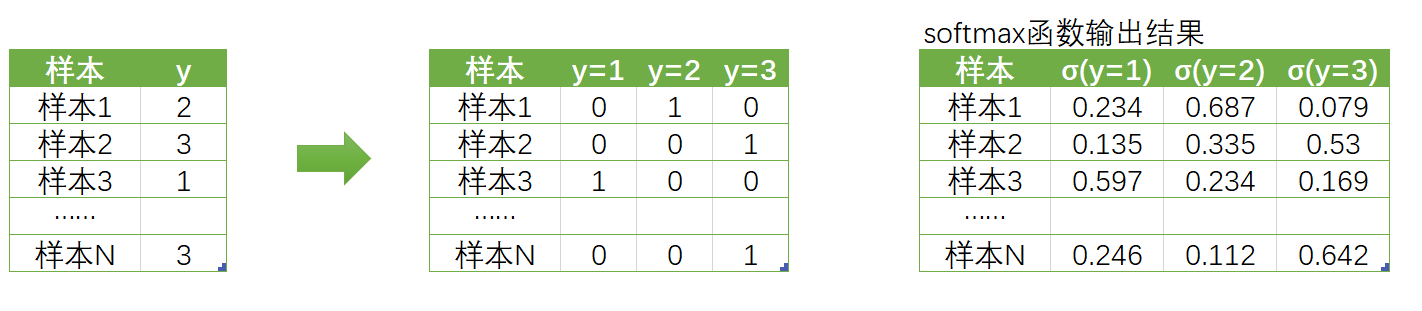原本的真实标签y是含有[1, 2, 3]三个分类的列向量，现在我们把它变成了标签矩阵，每个样本对应一个向量。（如果你熟悉机器学习或统计学，你能够一眼看出这其实就是独热编码one-hot）。在矩阵中，每一行依旧对应样本，但却由三分类衍生出了三个新的列，分别代表：真实标签是否等于1、等于2以及等于3。在矩阵中，我们使用“1”标注出样本的真实标签的位置，使用0表示样本的真实标签不是这个标签。不难注意到，这个标签矩阵的结构其实是和softmax函数输出的概率矩阵的结构一致，并且一一对应的。

回顾下二分类的似然函数：
P ( y ^ i ∣ x i , w ) = P 1 y i ∗ P 0 1 − y i P\left(\hat{y}_{i} \mid \boldsymbol{x}_{i}, \boldsymbol{w}\right)=P_{1}^{y_{i}} * P_{0}^{1-y_{i}}
当我们把标签整合为标签矩阵后，我们就可以将单个样本在总共K个分类情况整合为以下的似然函数：
P ( y ^ i ∣ x i , w ) = P 1 y i ( k = 1 ) ∗ P 2 y i ( k = 2 ) ∗ P 3 y i ( k = 3 ) ∗ … ∗ P K y i ( k = K ) P\left(\hat{y}_{i} \mid \boldsymbol{x}_{i}, \boldsymbol{w}\right)=P_{1}^{y_{i(k=1)}} * P_{2}^{y_{i(k=2)}} * P_{3}^{y_{i(k=3)}} * \ldots * P_{K}^{y_{i(k=K)}}
其中P就是样本标签被预测为某个具体值的概率，而右上角的指数就是标签矩阵中对应的值，即这个样本的真实标签是否为当前标签的判断（是就是1，否就是0）。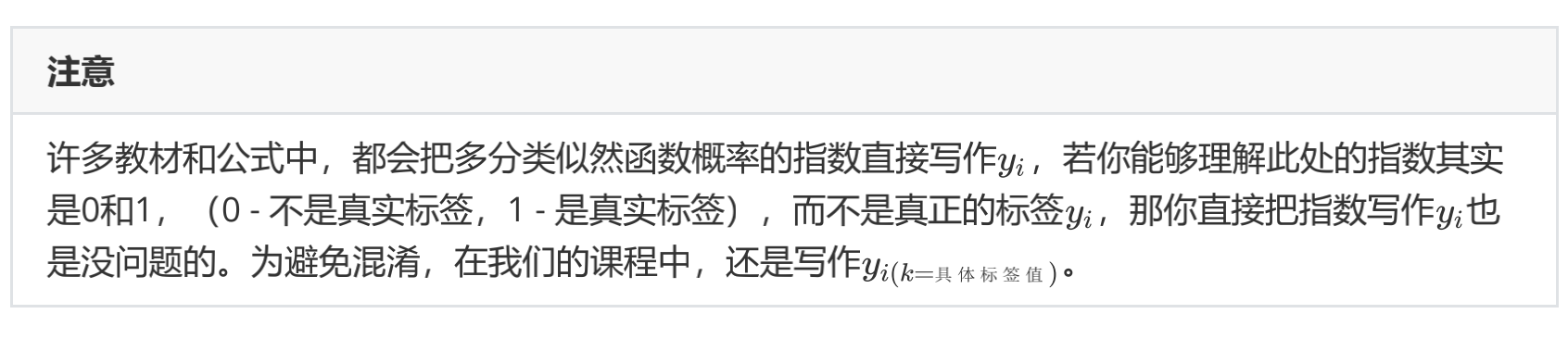更具体的，小k代表y的真实取值，K代表总共有K个分类（此处不是非常严谨，按道理说若K代表总共有K个类别，则不应该再使用K代表某个具体类别，但在这里，由于我们使用的类别编号与类别本身相同，所以为了公式的简化，使用了这样不严谨的表示方式）。虽然是连乘，但对于一个样本，除了自己所在的真实类别指数会是1之外，其他类别的指数都为0，所以被分类为其他类别的概率在这个式子里就都为0。所以我们可以将式子简写为：
P ( y ^ i ∣ x i , w ) = P j y i ( k = j ) , j  为样本  i  所对应的真实标签的编号  P\left(\hat{y}_{i} \mid \boldsymbol{x}_{i}, \boldsymbol{w}\right)=P_{j}^{y_{i(k=j)}}, j \text { 为样本 } i \text { 所对应的真实标签的编号 }
对一个训练集的m个样本来说，我们可以定义如下等式来表达所有样本在特征张量 X X 和权重向量 w w 组成的预测函数中，预测出所有可能的 y ^ \hat{y} 的概率P为：
P = ∏ i = 1 m P ( y ^ i ∣ x i , w ) = ∏ i = 1 m P j y i ( k = j ) = ∏ i = 1 m σ j y i ( k = j ) \begin{aligned} \boldsymbol{P} &=\prod_{i=1}^{m} P\left(\hat{y}_{i} \mid x_{i}, w\right) \\ &=\prod_{i=1}^{m} P_{j}^{y_{i(k=j)}} \\ &=\prod_{i=1}^{m} \sigma_{j}^{y_{i(k=j)}} \end{aligned}
这就是多分类状况下的似然函数。与二分类一致，似然函数解出来后，我们需要对似然函数求对数：
ln ⁡ P = ln ⁡ ∏ i = 1 m σ j y i ( k − j ) = ∑ i = 1 m ln ⁡ ( σ j y i ( k = j ) ) = ∑ i = 1 m y i ( k = j ) ln ⁡ σ i \begin{aligned} \ln \boldsymbol{P} &=\ln \prod_{i=1}^{m} \sigma_{j}^{y_{i(k-j)}} \\ &=\sum_{i=1}^{m} \ln \left(\sigma_{j}^{y_{i(k=j)}}\right) \\ &=\sum_{i=1}^{m} y_{i(k=j)} \ln \sigma_{i} \end{aligned}
这个函数就是我们之前提到过的交叉熵函数。不难看出，二分类的交叉熵函数其实是多分类的一种特殊情况。交叉熵函数十分特殊，虽然我们求解过程中，取对数的操作是在确定了似然函数后才进行的，但从计算结果来看，对数操作其实只对softmax函数的结果 σ \sigma 起效。因此在实际操作中，我们把 ln ⁡ ( softmax ⁡ ( z ) ) \ln (\operatorname{softmax}(z)) 这样的函数单独定义了一个功能做logsoftmax，PyTorch中可以直接通过nn.logsoftmax类调用这个功能。同时，我们把对数之外的，乘以标签、加和、取负等等过程打包起来，称之为负对数似然函数（Negative Log Likelihood function），在PyTorch中可以使用nn.NLLLoss来进行调用。也就是说，在计算损失函数时，我们不再需要使用单独的softmax函数了。

### 2 用PyTorch实现多分类交叉熵损失

在PyTorch中实现交叉熵函数的时候，有两种办法：

• 调用logsoftmax和NLLLoss实现
import torch
import torch.nn as nn
N = 3*pow(10,2)
torch.random.manual_seed(420)
X = torch.rand((N,4),dtype=torch.float32)
#定义y时应该怎么做？应该设置为矩阵吗？
y = torch.randint(low=0,high=3,size=(N,),dtype=torch.float32)
zhat = torch.mm(X,w) #从这里开始调用softmax和NLLLoss

logsm = nn.LogSoftmax(dim=1) #实例化
logsigma = logsm(zhat)

criterion = nn.NLLLoss() #实例化
#由于交叉熵损失需要将标签转化为独热形式，因此不接受浮点数作为标签的输入
#对NLLLoss而言，需要输入logsigma
criterion(logsigma,y.long()) #y一维、整型


更加简便的方法是：

• 直接调用CrossEntropyLoss
criterion = nn.CrossEntropyLoss()
criterion(zhat,y.long()) #一维、整型


可以发现，两种输出方法得到的损失函数结果是一致的。与其他损失函数一致，CrossEntropyLoss也有参数reduction，可以设置为mean、sum以及None，大家可以自行尝试其代码并查看返回结果。

无论时二分类还是多分类，PyTorch都提供了包含输出层激活函数和不包含输出层激活函数的类两种选择。在实际神经网络建模中，类可以被放入定义好的Model类中去构建神经网络的结构，因此是否包含激活函数，就需要由用户来自行选择。

• 重视展示网络结构和灵活性，应该使用不包含输出层激活函数的类

通常在Model类中，__init__中层的数量与forward函数中对应的激活函数的数量是一致的，如果我们使用内置sigmoid/logsoftmax功能的类来计算损失函数，forward函数在定义时就会少一层（输出层），网络结构展示就不够简单明了，对于结构复杂的网络而言，结构清晰就更为重要。同时，如果激活函数是单独写的，要修改激活函数就变得很容易，如果混在损失函数中，要修改激活函数时就得改掉整个损失函数的代码，不利于维护。

• 重视稳定性和运算精度，使用包含输出层激活函数的类

如果在一个Model中，很长时间我们都不会修改输出层的激活函数，并且模型的稳定运行更为要紧，我们就使用内置了激活函数的类来计算损失函数。同时，就像之前提到的，内置激活函数可以帮助我们推升运算的精度。

因此，选择哪种损失函数的实现方式，最终还要看我们的需求。

有了损失函数，我们终于要开始进行求解了。下一部分我们来讲解神经网络的入门级优化算法：小批量随机梯度下降。

展开全文神经网络 机器学习
• 信息熵： 表示随机变量不确定的度量，是对所有可能发生的事件产生的信息...交叉熵(cross entropy)： 将KL散度公式进行变形得到： 前半部分就是p(x)的熵，后半部分就是交叉熵： 机器学习中，我们常常使用KL散度来评估pr
• 理解交叉熵关于样本集的两个概率分布p和q，设p为真实的分布，比如[1, 0, 0]表示当前样本属于第一类，q为拟合的分布，比如[0.7, 0.2, 0.1]。按照真实分布p来衡量识别一个样本所需的编码长度的期望，即平均编码长度...

理解交叉熵

关于样本集的两个概率分布p和q，设p为真实的分布，比如[1, 0, 0]表示当前样本属于第一类，q为拟合的分布，比如[0.7, 0.2, 0.1]。

按照真实分布p来衡量识别一个样本所需的编码长度的期望，即平均编码长度(信息熵)：如果使用拟合分布q来表示来自真实分布p的编码长度的期望，即平均编码长度(交叉熵)：直观上，用p来描述样本是最完美的，用q描述样本就不那么完美，根据吉布斯不等式，恒成立，当q为真实分布时取等，我们将由q得到的平均编码长度比由p得到的平均编码长度多出的bit数称为相对熵，也叫KL散度：在机器学习的分类问题中，我们希望缩小模型预测和标签之间的差距，即KL散度越小越好，在这里由于KL散度中的项不变(在其他问题中未必)，故在优化过程中只需要关注交叉熵就可以了，因此一般使用交叉熵作为损失函数。

多分类任务中的交叉熵损失函数其中是一个概率分布，每个元素表示样本属于第i类的概率；是样本标签的onehot表示，当样本属于第类别i时，否则;c是样本标签。

PyTorch中的交叉熵损失函数实现

PyTorch提供了两个类来计算交叉熵，分别是CrossEntropyLoss() 和NLLLoss()。torch.nn.CrossEntropyLoss()

类定义如下

torch.nn.CrossEntropyLoss(

weight=None,

ignore_index=-100,

reduction="mean",

)表示一个样本的非softmax输出，c表示该样本的标签，则损失函数公式描述如下，如果weight被指定，其中，import torch

import torch.nn as nn

model = nn.Linear(10, 3)

criterion = nn.CrossEntropyLoss()

x = torch.randn(16, 10)

y = torch.randint(0, 3, size=(16,)) # (16, )

logits = model(x) # (16, 3)

loss = criterion(logits, y)torch.nn.NLLLoss()

类定义如下

torch.nn.NLLLoss(

weight=None,

ignore_index=-100,

reduction="mean",

)表示一个样本对每个类别的对数似然(log-probabilities)，c表示该样本的标签，损失函数公式描述如下，其中，import torch

import torch.nn as nn

model = nn.Sequential(

nn.Linear(10, 3),

nn.LogSoftmax()

)

criterion = nn.NLLLoss()

x = torch.randn(16, 10)

y = torch.randint(0, 3, size=(16,)) # (16, )

out = model(x) # (16, 3)

loss = criterion(out, y)总结

torch.nn.CrossEntropyLoss在一个类中组合了nn.LogSoftmax和nn.NLLLoss，This criterion combines nn.LogSoftmax() and nn.NLLLoss()in one single class. The input is expected to contain scores for each class.

展开全文• mse均方误差、mae绝对值平均误差用于拟合回归，公式已经熟悉了，但交叉熵的每次都只是应用，没有了解公式，这对于自己写交叉熵损失函数以及分析损失函数不利。 公式详解 C是损失值； n是求平均用的，所以是样本数量...

# 背景

mse均方误差、mae绝对值平均误差用于拟合回归，公式已经熟悉了，但交叉熵的每次都只是应用，没有了解公式，这对于自己写交叉熵损失函数以及分析损失函数不利。

# 公式详解

C是损失值；
n是求平均用的，所以是样本数量，也就是batchsize；
x是预测向量维度，因为需要在输出的特征向量维度上一个个计算并求和；
y是onehot编码后的真实值 对应x维度上的标签，是1或0；
a是onehot格式输出的预测标签，是0~1的值，a经过了softmax激活，所以a的和值为1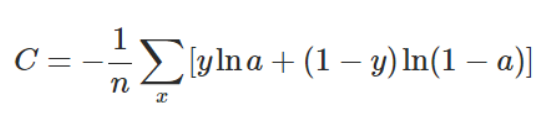对于某个维度 x i x_i y = 1 y=1 时a越大越好，相反a越小越好，C值为两者和的负值，所以越好→C↓ 所以可以最优化C（神经网络需要最小化损失函数）

# 公式计算举例

C ( ( 0.8 , 0.1 , 0.1 ) , ( 1 , 0 , 0 ) ) = − 1 / 1 ∗ ( 1 l n ( 0.8 ) + 0 + 0 + 1 l n ( 0.9 ) + 0 + 1 l n ( 0.9 ) ) C((0.8,0.1,0.1),(1,0,0))=-1/1*(1ln(0.8)+0+0+1ln(0.9)+0+1ln(0.9))

# 公式编程实现

计算举例是为了理解计算过程，最终还是要落到编程实现上：

def cross_entropy(y_true,y_pred):
C=0
# one-hot encoding
for col in range(y_true.shape[-1]):
y_pred[col] = y_pred[col] if y_pred[col] < 1 else 0.99999
y_pred[col] = y_pred[col] if y_pred[col] > 0 else 0.00001
C+=y_true[col]*np.log(y_pred[col])+(1-y_true[col])*np.log(1-y_pred[col])
return -C

# 没有考虑样本个数 默认=1
num_classes = 3
label=1#设定是哪个类别 真实值

y_true = np.zeros((num_classes))
# y_pred = np.zeros((num_classes))
# preset
y_true[label]=1
y_pred = np.array([0.0,1.0,0.0])
C = cross_entropy(y_true,y_pred)
print(y_true,y_pred,"loss:",C)
y_pred = np.array([0.1,0.8,0.1])
C = cross_entropy(y_true,y_pred)
print(y_true,y_pred,"loss:",C)
y_pred = np.array([0.2,0.6,0.2])
C = cross_entropy(y_true,y_pred)
print(y_true,y_pred,"loss:",C)
y_pred = np.array([0.3,0.4,0.3])
C = cross_entropy(y_true,y_pred)
print(y_true,y_pred,"loss:",C)


执行结果：

[0. 1. 0.] [1.0000e-05 9.9999e-01 1.0000e-05] loss: 3.0000150000863473e-05
[0. 1. 0.] [0.1 0.8 0.1] loss: 0.43386458262986227
[0. 1. 0.] [0.2 0.6 0.2] loss: 0.9571127263944101
[0. 1. 0.] [0.3 0.4 0.3] loss: 1.62964061975162

Process finished with exit code 0


# 结论

1. 分类任务神经网络的输出层往往是经过了softmax激活，所以最后一层输出的预测向量各个维度的值均为0~1范围内的数。
2. python的引用类型，如array，在函数传参后是传的引用，所以函数内的修改会影响到实际的值，通过控制台输出的第一条信息即可知。
3. 计算过程可能出现溢出NaN的报错，所以需要进行近似处理。
展开全文神经网络 python 深度学习 人工智能 算法
• 腾讯一面 面试题 那时候研一上学期刚结束 还不太扎实 勉勉强强算了一遍，这里重新整理下 首先写出多分类交叉熵损失函数公式： 那么 y j y_j yj​是真实标签向量one-hot表示， a j a_j aj​是模型输出标签向量表示。...自然语言处理
• 一、Softmax函数的导数 Softmax函数公式： Si 代表的是第i个神经元的输出 其中wij 是第i个神经元的第 ...多分类损失函数公式： 其中yi表示真实的分类结果 利用损失函数求梯度 已知不做推导 求解书过程推导： 如果i自然语言处理 神经网络 深度学习
• 分类问题的交叉熵损失函数推导与二分类交叉熵损失函数推导的思想是一致的。可以先参考 二分类问题的交叉熵损失函数推导可参考二分类问题的交叉熵损失函数推导。 本文参考deeplearning 一书 6.2.2.3 Softmax Units...softmax 机器学习 人工智能
• 本代码基于pytorch实现，复现ICCV 2019论文 Robust Loss Functions under Label Noise for Deep Neural Networks，论文地址https://arxiv.org/abs/1712.09482v1
• 它与二进制分类的代码相同，但当我添加另一个类并将compile方法的丢失函数更改为使用category\u crossentry时，会出现以下错误(=&gt；在代码末尾)。有人能解释一下这是什么问题吗？我犯了什么错误？在# ...
• 误差平方和SSE三、二分类交叉熵损失函数1 极大似然估计求解二分类交叉熵损失2 用tensor实现二分类交叉熵损失3 用PyTorch中的类实现二分类交叉熵损失四、多分类交叉熵损失函数 一、机器学习中的优化思想 模型训练的...pytorch 神经网络 深度学习
• 分类问题的交叉熵损失函数;在二分类问题中，损失函数为交叉熵损失函数。对于样本(x,y)来讲，x为样本 y为对应的标签。...多分类的问题的函数交叉熵损失函数：在多分类问题中，损失函数也是交叉熵损...
• 写在前面 在文章[TensorFlow] argmax, softmax_cross_entropy_with_logits, sparse_softmax_cross_entropy_with_logits函数...当存在个类别时，通常使用交叉熵损失函数来衡量模型的效果，也是对模型调参的重要依据，tensorflow 深度学习
• 说起交叉熵损失函数「Cross Entropy Loss」，相信大家都非常熟悉，但是要深入理解交叉熵损失函数的原理和作用，还得溯本追源才能对其有一个真实的理解与认知。 交叉熵 交叉熵是用来度量两个概率分布的差异性的，...信息熵 机器学习ML
• tf.keras.losses.CategoricalCrossentropy 多分类损失函数示例 文章目录tf.keras.losses.CategoricalCrossentropy 多分类损失函数示例计算公式tf.keras.losses.CategoricalCrossentropy 实现numpy 实现 import numpy...keras tensorflow
• ## 交叉熵损失函数

千次阅读 2020-12-19 05:17:03
交叉熵代价函数(Cross-entropy cost function)是用来衡量人工神经网络(ANN)的预测值与实际值的一种方式。与二次代价函数相比，它能更有效地促进ANN的训练。在介绍交叉熵代价函数之前，本文先简要介绍二次代价函数，...
• 通过矩阵变换，将最后的输出值定为1维0 1之间的数值，再用BCEloss函数（二分类交叉熵损失函数）构建计算图 分类： 隐藏层用激活sigmoid函数处理线性变换后的值，最后一层用softmax函数【e（x）/∑e（x）】算出输出...神经网络 pytorch 深度学习
• 之前在代码中经常看见交叉熵损失函数(CrossEntropy Loss)，只知道它是分类问题中经常使用的一种损失函数，对于其内部的原理总是模模糊糊，而且一般使用交叉熵作为损失函数时，在模型的输出层总会接一个softmax函数，...
• 进行二分类多分类问题时，在众多损失函数中交叉熵损失函数较为常用。 下面的内容将以这三个问题来展开 写目录标题什么是交叉熵损失以图片分类问题为例，理解交叉熵损失函数从0开始实现交叉熵损失函数 什么是交叉熵...机器学习
• 目录0. 前言1.损失函数（Loss Function）1.1 损失项1.2 正则化项2....今天在学习cs231n的时候看到了关于交叉熵损失函数的讲解，发现之前虽然经常用到这个损失函数，但却对里面的细节很模糊，学完之后更清晰了一神经网络 机器学习 深度学习 正则化
• 解决多分类问题有几种思路。有将多分类问题拆分成一...我们自然地从类别逻辑回归公式中推导出交叉熵损失函数，理解交叉熵损失函数的由来。一、类别逻辑回归( Multinomial logistic regression )我们之间讲解了...
• 详细介绍了交叉熵损失函数，包括二分类交叉熵损失函数多分类交叉熵损失函数，并对其分类损失的举例计算；详细介绍了softmax函数。深度学习...